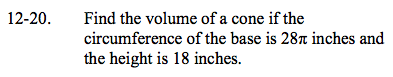### Home > GC > Chapter 12 > Lesson 12.1.2 > Problem12-20

12-20.The formula for the circumference of a circle is 2πr.

$\text{The formula for the volume of a cone is }\frac{base \cdot height}{3}.$

28 = 2r

28π = 2πr

r = 14

Base = r²π
= 14²π
= 196π

≈ 3694.5 in³

$\text{Volume}=\frac{196\pi \cdot 18}{3}$

$=\frac{3528\pi}{3}=1176\pi$